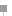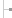Working with variables in Formula language for Lotus Notes

Learn about the basic syntax rules for working with variables in Formula language for Lotus Notes.

The following is tip #2 from "A Formula language for Lotus Notes introduction -- 7 tips in 7 minutes," excerpted from Chapter 5 of the book Lotus Notes Developer's Toolbox , published by IBM Publishing.

Working with variables

Now let's take a look at the basic syntax rules for working with variables. There are two types of variables that can be utilized in formulas -- temporary variables and field name variables.

Temporary variables, as the name implies, are used to temporarily store a value associated with the formula. Temporary variables cannot be referenced outside of the formula and only contain a value for the duration of the formula's execution. In other words, the value stored in a temporary variable is not saved when the document or form is saved and cannot be referenced by other formulas.

The syntax for a creating a temporary variable is VariableName and the assignment operator := followed by the data value and semicolon. For example, the following assigns "January" to the temporary variable called month.

month := "January";

Conversely, field name variables reference actual fields on a document or form. When used in a formula, you can reference or change the value stored in the field name. In other words, by using formulas, you can use field names to calculate the main expression or modify the value already stored in the field.

There are three ways to reference an existing field. The FIELD keyword is used to assign a value to an existing field. Using this keyword, the contents of the field are permanently changed and will be stored in the field when the document is saved. The syntax for setting a field value in a form or document is FIELD FieldName and the assignment operator := followed by the new value and a semicolon. For example:

FIELD Month := "January";

It's important to note that if the field exists on the form, the new value will replace the existing data value. If the field does not exist on the form, Notes will create the field and assign the value. Alternatively, you can also use the @SetField function. This function works just like FIELD with one exception: @SetField can be imbedded within other Formula language functions.

@SetField ("Month"; "January");

Finally, to acquire the value of an existing field, you can use the @GetField function. Using this function, you can use the value stored in field inside another formula. For example, the following will create the text string "Your project is due in: January," assuming the Month field contains a value of "January".

"Your project is due in: " + @GetField ( "Month" );

NOTE:

Lotus Notes will recognize a variable name regardless of the letter case. Variable names can be uppercase, lowercase, or mixed case and will still be understood. For example, the following are equivalent variable names: firstname, FIRSTNAME, FirstName, and FIRSTname.A Formula language for Lotus Notes introductionHome: IntroductionTip 1: What is Lotus Formula Language?Tip 2: Working with variables in Formula language for Lotus NotesTip 3: Lotus Notes Formula language keywordsTip 4: Working with operators in Formula language for Lotus NotesTip 5: Lotus Notes Formula language general syntax rulesTip 6: What are Lotus Notes Formula language functions and commands?Tip 7: Working with text strings in Formula language for Lotus NotesThis chapter excerpt from Lotus Notes Developer's Toolbox, by Mark , is printed with permission from IBM Publishing, Copyright 2006. Click here for the chapter download or purchase the book here.

Dig Deeper on Lotus Notes 5

• Favorite iSeries cheat sheets

Here you'll find a collection of valuable cheat sheets gathered from across the iSeries/Search400.com community. These cheat ...

Close